# Highlight the Dates if its over a year

This post will guide you how to highlight the dates that are over 1 year in excel. How do I highlight the dates over a year old using conditional formatting feature in excel. How to use conditional formatting to highlight if the dates are over a year in excel. How to highlight cells color when the date is over a year old in excel.

## Highlight the Date that Are over 1 year

Assuming that you have a list of date in the range of cells B1:B5, and you would like to make it easy to determine all dates which are older than 1 year, then highlight it. You can use the Conditional formatting feature to highlight the dates over a year old, just do the following steps:

#1 select the range of cells that contain the dates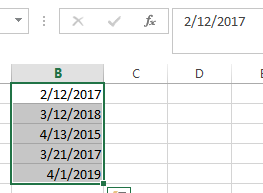#2 go to HOME tab, click Conditional Formatting command under Styles group, then select the New Rule… from the drop-down menu list. The New Formatting Rule dialog will appear.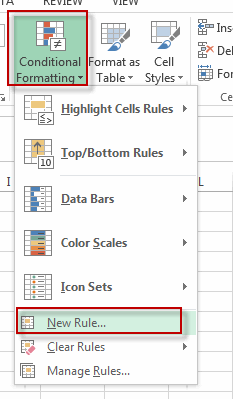#3 select Use a formula to determine which cells to format in the Select a Rule type list, and type the following formula =B1<=TODAY()-365 into the textbox of the Format values where this formula is true.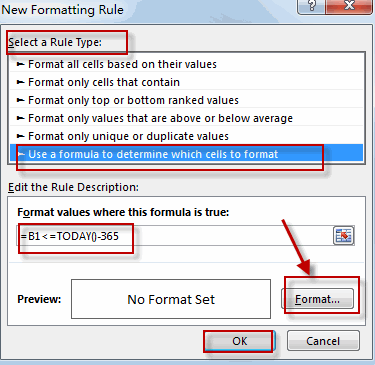#4 click Format button, the Format Cells dialog will appear.

#5 switch Fill tab, select one color that you want to highlight the dates. Click OK button.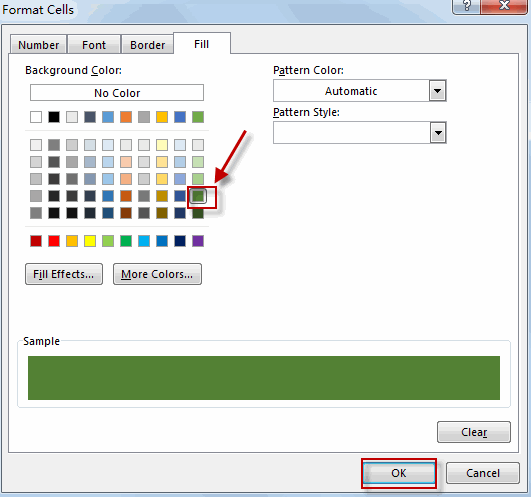#6 let’s see the result: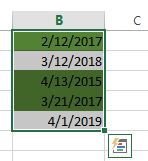You will see that all the dates over a year have been highlighted with a specified color in the range of cells B1:B5.

Related Posts

Calculate Cumulative Totals with Excel SUM Function

Today, through a simple example, we will show you how to use one of the most common-used Mathematical functions in excel, the SUM function, to add up the sum. In our daily life, we keep an account of what we ...

BMI Calculation Formula In Ms Excel

You might have come across a task in which you were assigned to make BMI calculations of the supplied numbers, and you may be looking for an efficient approach to accomplish this process rather than doing BMI calculations manually, by ...

Calculate The Period of Loan or Investment in Excel

In our daily life, most of us will invest or take a loan, so we need to master some simple financial functions to calculate the period needed for loans or investments. If you work as an accountant, you need to ...

Working Time Calculation Based on Timesheets

In the office, a special machine record the time when you start working (clock in office) and when you finish working (clock out of office). We can calculate the total working time by subtracting the end working time from the ...

Calculate Days Open in Excel

If you want to know how to Calculate days in Excel, there are some formulas that you can use to do so. For example, you can use the DAYS function in Excel to find the number of days between two ...

How to Sum Last N Days in Excel

In our daily life, we may want to sum amounts or sales for a specific period, for example in last N days. Sum numbers in Excel is easy to run, we can apply SUM function. But if we want to ...

How to Calculate Retirement Date and Remaining Years refer to Birth Date in Excel

Every company has its own police about the retirement date for employees. Suppose employees will be retired from a certain age of 60, how can we calculate the retirement date for everyone base on their birthdays? Actually, there are several ...

How to Sum If Date is Greater or Less Than in Excel

If we sum for certain conditions, we'll use the SUMIF function in excel. For example, count the total sales for a period before of after a specified date. This article will help you to do sum for a specified period ...

How to Highlight Cell or Row If Date Is In Current Day/Week/Month in Excel

This post will guide you how to highlight cell if date is the current day or is in the current week or month in Excel. How do I highlight row if date is in current week or month with conditional ...

How to Add the Current Month or Year in a Cell in Excel

This post will guide you how to add the current month or year into a cell or header or footer in your worksheet. How do I insert the current date or time in a cell with a formula in Excel. ...

Sidebar